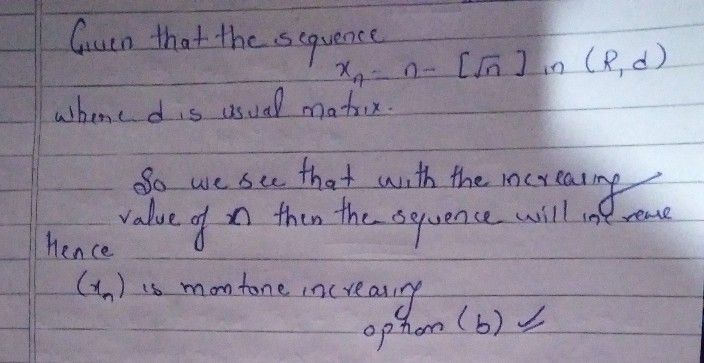Symbol
Problem$\left(12\right)$ Consider the sequence $x_{n}=n-\left(\sqrt{n}$ $in\left(\bar{F}$ (R, $d\right)$ where $d$ is usual metric. Then $\left($ (a) $a\right)$ $\left(x_{n}\right)$ is Cauchy. $\left($ (b) $\left(x_{n}\right)$ is monotone increasing. $\left($ (c) $\left(x_{n}\right)$ is monotone decreasing. $\left($ $d\right)$ $\left(x_{n}\right)$ is not convergent but has a convergent subsequence.
Other
Search count: 119
SolutionQanda teacher - priya